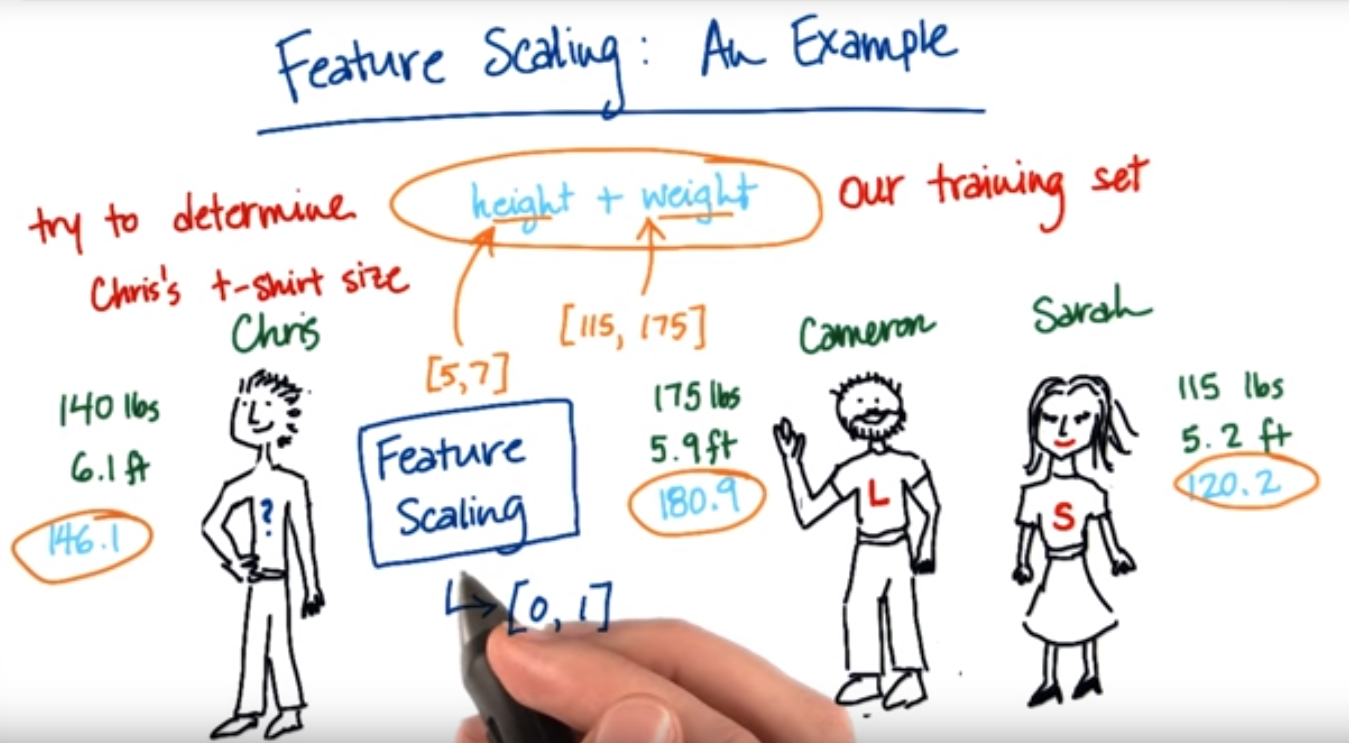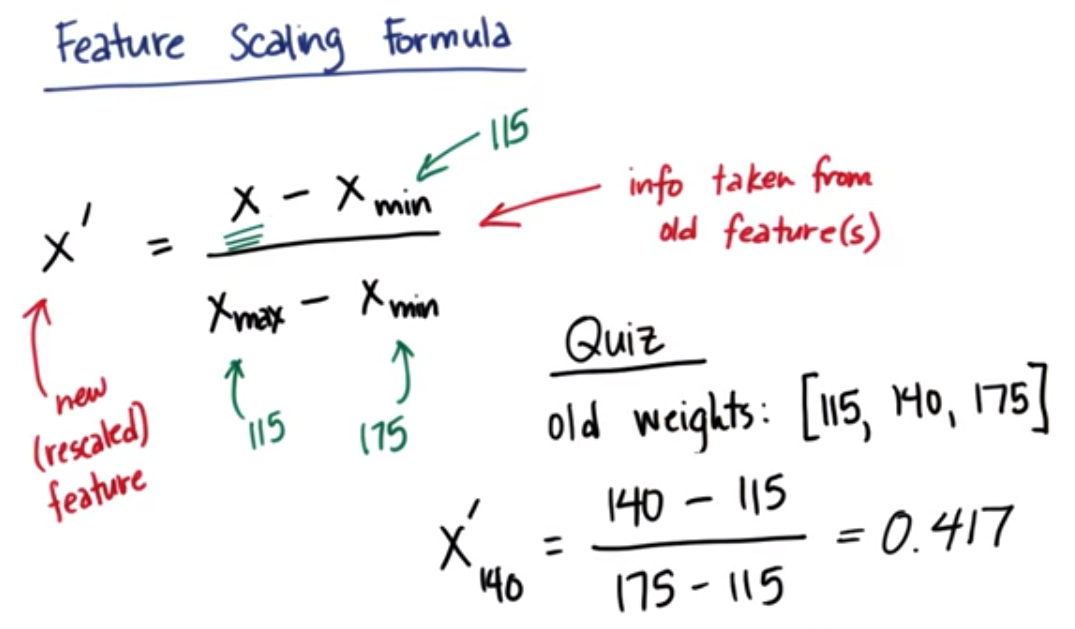# 6. 利用不同的尺度来比较特征# 9. 特征缩放公式练习# 10. 最小值/最大值重缩放器编码练习

``````def featureScaling(arr):
arr.sort()

# 避免所有值都是相同的情况
if arr == arr[-1]:
return 1

new_arr = []

for i in arr:
new_data = float(i-arr)/(arr[-1]-arr)
new_arr.append(new_data)

return new_arr

# tests of your feature scaler--line below is input data
data = [115, 140, 175]
print featureScaling(data)
``````

``````[0.0, 0.4166666666666667, 1.0]
Good job! Your output matches our solution.
``````

# 11. sklearn 中的最小值/最大值缩放器

``````>>> from sklearn.preprocessing import MinMaxScaler
>>> data = [[-1, 2], [-0.5, 6], [0, 10], [-3, 18]]
>>> scaler = MinMaxScaler()
>>> print scaler.fit(data)
MinMaxScaler(copy=True, feature_range=(0, 1))
>>>
>>> print scaler.data_max_
[  0.  18.]
>>> print scaler.data_min_
[-3.  2.]

>>> print scaler.transform(data)
[[ 0.    0.  ]
[ 0.25  0.25]
[ 0.5   0.5 ]
[ 1.    1.  ]]
>>>
>>> print scaler.transform([[2,2]])
[[ 1.5  0. ]]
``````

# 12. 需要重缩放的算法练习

• 决策树
• [★影响]使用 RBF 核函数的 SVN
• 线性回归
• [★影响]K-均值聚类